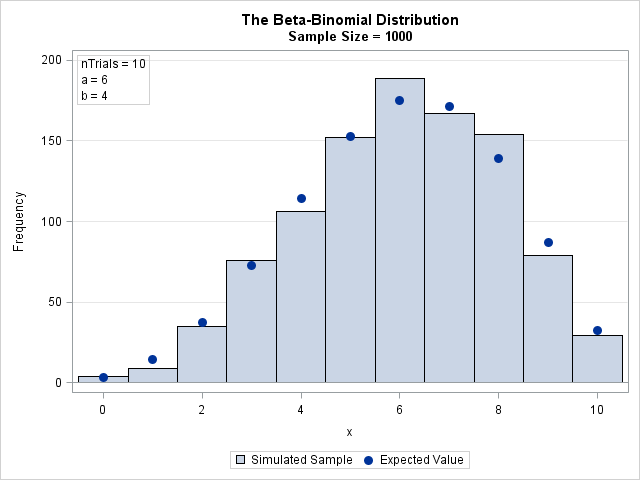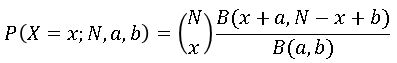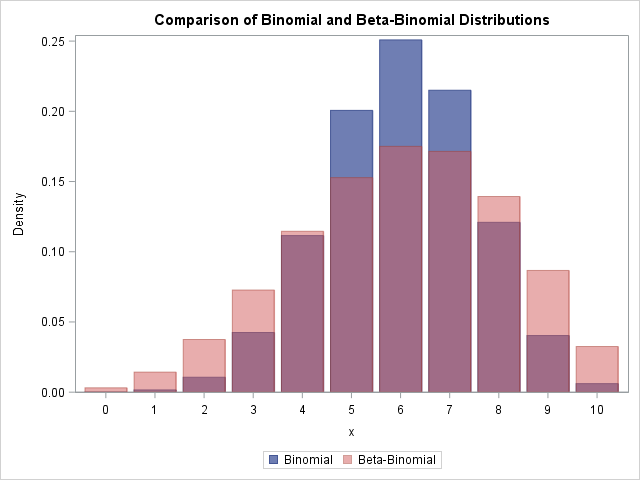This article shows how to simulate beta-binomial data in SAS and how to compute the density function (PDF). The beta-binomial distribution is a discrete compound distribution. The "binomial" part of the name means that the discrete random variable X follows a binomial distribution with parameters N (number of trials) and p, but there is a twist: The parameter p is not a constant value but is a random variable that follows the Beta(a, b) distribution.

The beta-binomial distribution is used to model count data where the counts are "almost binomial" but have more variance than can be explained by a binomial model. Therefore this article also compares the binomial and beta-binomial distributions.

### Simulate data from the beta-binomial distribution

To generate a random value from the beta-binomial distribution, use a two-step process. The first step is to draw p randomly from the Beta(a, b) distribution. Then you draw x from the binomial distribution Bin(p, N). The beta-binomial distribution is not natively supported by the RAND function SAS, but you can call the RAND function twice to simulate beta-binomial data, as follows:

```/* simulate a random sample from the beta-binomial distribution */ %let SampleSize = 1000; data BetaBin; a = 6; b = 4; nTrials = 10; /* parameters */ call streaminit(4321); do i = 1 to &SampleSize; p = rand("Beta", a, b); /* p[i] ~ Beta(a,b) */ x = rand("Binomial", p, nTrials); /* x[i] ~ Bin(p[i], nTrials) */ output; end; keep x; run;```

The result of the simulation is shown in the following bar chart. The expected values are overlaid. The next section shows how to compute the expected values.### The PDF of the beta-binomial distribution

The Wikipedia article about the beta-binomial distribution contains a formula for the PDF of the distribution. Since the distribution is discrete, some references prefer to use "PMF" (probability mass function) instead of PDF. Regardless, if X is a random variable that follows the beta-binomial distribution then the probability that X=x is given bywhere B is the complete beta function.

The binomial coefficients ("N choose x") and the beta function are defined in terms of factorials and gamma functions, which get big fast. For numerical computations, it is usually more stable to compute the log-transform of the quantities and then exponentiate the result. The following DATA step computes the PDF of the beta-binomial distribution. For easy comparison with the distribution of the simulated data, the DATA step also computes the expected count for each value in a random sample of size N. The PDF and the simulated data are merged and plotted on the same graph by using the VBARBASIC statement in SAS 9.4M3. The graph was shown in the previous section.

```data PDFBetaBinom; /* PMF function */ a = 6; b = 4; nTrials = 10; /* parameters */ do x = 0 to nTrials; logPMF = lcomb(nTrials, x) + logbeta(x + a, nTrials - x + b) - logbeta(a, b); PMF = exp(logPMF); /* probability that X=x */ EX = &SampleSize * PMF; /* expected value in random sample */ output; end; keep x PMF EX; run;   /* Merge simulated data and PMF. Overlay PMF on data distribution. */ data All; merge BetaBin PDFBetaBinom(rename=(x=t)); run;   title "The Beta-Binomial Distribution"; title2 "Sample Size = &SampleSize"; proc sgplot data=All; vbarbasic x / barwidth=1 legendlabel='Simulated Sample'; /* requires SAS 9.4M3 */ scatter x=t y=EX / legendlabel='Expected Value' markerattrs=GraphDataDefault(symbol=CIRCLEFILLED size=10); inset "nTrials = 10" "a = 6" "b = 4" / position=topleft border; yaxis grid; xaxis label="x" integer type=linear; /* force TYPE=LINEAR */ run;```

### Compare the binomial and beta-binomial distributions

One application of the beta-binomial distribution is to model count data that are approximately binomial but have more variance ("thicker tails") than the binomial model predicts. The expected value of a Beta(a, b) distribution is a/(a + b), so let's compare the beta-binomial distribution to the binomial distribution with p = a/(a + b).

The following graph overlays the two PDFs for a = 6, b = 4, and nTrials = 10. The blue distribution is the binomial distribution with p = 6/(6 + 4) = 0.6. The pink distribution is the beta-binomial. You can see that the beta-binomial distribution has a shorter peak and thicker tails than the corresponding binomial distribution. The expected value for both distributions is 6, but the variance of the beta-binomial distribution is greater. Thus you can use the beta-binomial distribution as an alternative to the binomial distribution when the data exhibit greater variance than expected under the binomial model (a phenomenon known as overdispersion).### Summary

The beta-binomial distribution is an example of a compound distribution. You can simulate data from a compound distribution by randomly drawing the parameters from some distribution and then using those random parameters to draw the data. For the beta-binomial distribution, the probability parameter p is drawn from a beta distribution and then used to draw x from a binomial distribution where the probability of success is the value of p. You can use the beta-binomial distribution to model data that have greater variance than expected under the binomial model.

The post Simulate data from the beta-binomial distribution in SAS appeared first on The DO Loop.